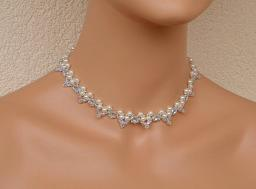# President's 5862

For the opening ball of the season, the president's wife bought a necklace with a bracelet for 768 euros. How much did the necklace cost, and how much did the bracelet cost if the bracelet was 40% off

a) cheaper,
b) more expensive than the necklace?

a =  448
b =  320
c =  320
d =  448

### Step-by-step explanation:

a+b = 768
a = b + 40/100 b
c+d = 768
d = c + 40/100 c

a+b = 768
a = b + 40/100·b
c+d = 768
d = c + 40/100·c

a+b = 768
100a-140b = 0
c+d = 768
140c-100d = 0

Pivot: Row 1 ↔ Row 2
100a-140b = 0
a+b = 768
c+d = 768
140c-100d = 0

Row 2 - 1/100 · Row 1 → Row 2
100a-140b = 0
2.4b = 768
c+d = 768
140c-100d = 0

Pivot: Row 3 ↔ Row 4
100a-140b = 0
2.4b = 768
140c-100d = 0
c+d = 768

Row 4 - 1/140 · Row 3 → Row 4
100a-140b = 0
2.4b = 768
140c-100d = 0
1.7143d = 768

d = 768/1.71428571 = 448
c = 0+100d/140 = 0+100 · 448/140 = 320
b = 768/2.4 = 320
a = 0+140b/100 = 0+140 · 320/100 = 448

a = 448
b = 320
c = 320
d = 448

Our linear equations calculator calculates it.Did you find an error or inaccuracy? Feel free to write us. Thank you!

Tips for related online calculators